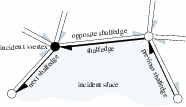## CGAL::Nef_polyhedron_S2<Traits>::SHalfedge

### Definition

A shalfedge is a great arc on a sphere map. The figure below depicts the relationship between a shalfedge and its incident shalfedges, svertices, and sfaces on a sphere map. A shalfedge is an oriented sedge between two svertices. It is always paired with a shalfedge pointing in the opposite direction. The twin() member function returns this shalfedge of opposite orientation.Figure:

The snext() member function points to the successor shalfedge around this sface while the sprev() member function points to the preceding shalfedge. An successive assignments of the form se = se->snext() cycles counterclockwise around the sface (or hole).

Similarly, the successive assignments of the form se = se->snext()->twin() cycle clockwise around the svertex and traverse all halfedges incident to this svertex. The assignment se = se->cyclic_adj_succ() can be used as a shortcut.

A const circulator is provided for each of the two circular orders. The circulators are bidirectional and assignable to SHalfedge_const_handle.

#include <CGAL/Nef_polyhedron_S2.h>

### Types

The following types are the same as in Nef_polyhedron_S2<Traits>.

 Nef_polyhedron_S2::SHalfedge::Mark type of mark. Nef_polyhedron_S2::SHalfedge::Sphere_circle sphere circle type stored in SHalfedge. Nef_polyhedron_S2::SHalfedge::SVertex_const_handle const handle to SVertex. Nef_polyhedron_S2::SHalfedge::SHalfedge_const_handle const handle to SHalfedge. Nef_polyhedron_S2::SHalfedge::SFace_const_handle const handle to SFace.

### Creation

There is no need for a user to create a SHalfedge explicitly. The class Nef_polyhedron_S2<Traits> manages the needed shalfedges internally.

### Operations

 Mark se.mark () the mark of se . Sphere_circle se.circle () the sphere circle of se . SHalfedge_const_handle se.twin () the twin of se . SVertex_const_handle se.source () the source svertex of se . SVertex_const_handle se.target () equals twin()->source(). SHalfedge_const_handle se.sprev () the SHalfedge previous to se in a sface cycle. SHalfedge_const_handle se.snext () the next SHalfedge of se in a sface cycle. SHalfedge_const_handle se.cyclic_adj_pred () the edge before se in the cyclic ordered adjacency list of source(). SHalfedge_const_handle se.cyclic_adj_succ () the edge after se in the cyclic ordered adjacency list of source(). SFace_const_handle se.incident_sface () the incident sface of se .